maths > fractions

Multiplication of fractions

what you'll learn...

overview

•  multiplication in first principles -- repeatedly combining a quantity and measuring the combined and

•  simplified procedure : Multiplying numerators and denominators

repeated times

Multiplication of integers or whole numbers: $4×3=12$$4 \times 3 = 12$

•  $4$$4$ is the multiplicand

•  $3$$3$ is the multiplier

•  $12$$12$ is the product

Multiplication is repeating the multiplicand the multiplier times -- to get the product.
$4$$4$ repeated $3$$3$ times =
$4+4+4=12$$4 + 4 + 4 = 12$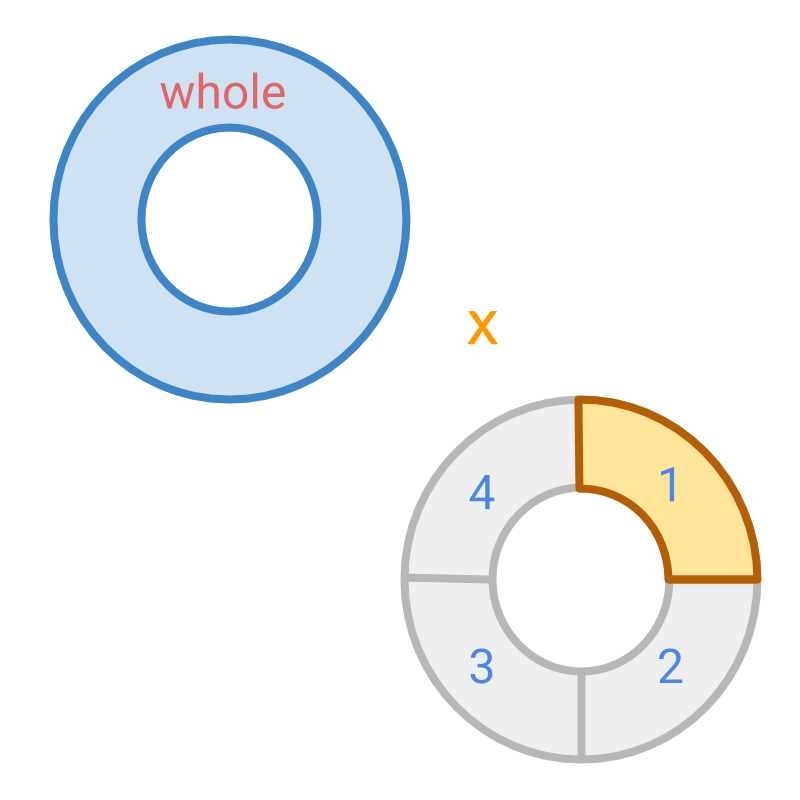Multiplication by a fraction : Consider $1×\frac{1}{4}$$1 \times \frac{1}{4}$
Multiplicand $1$$1$ is multiplied by a multiplier $\frac{1}{4}$$\frac{1}{4}$.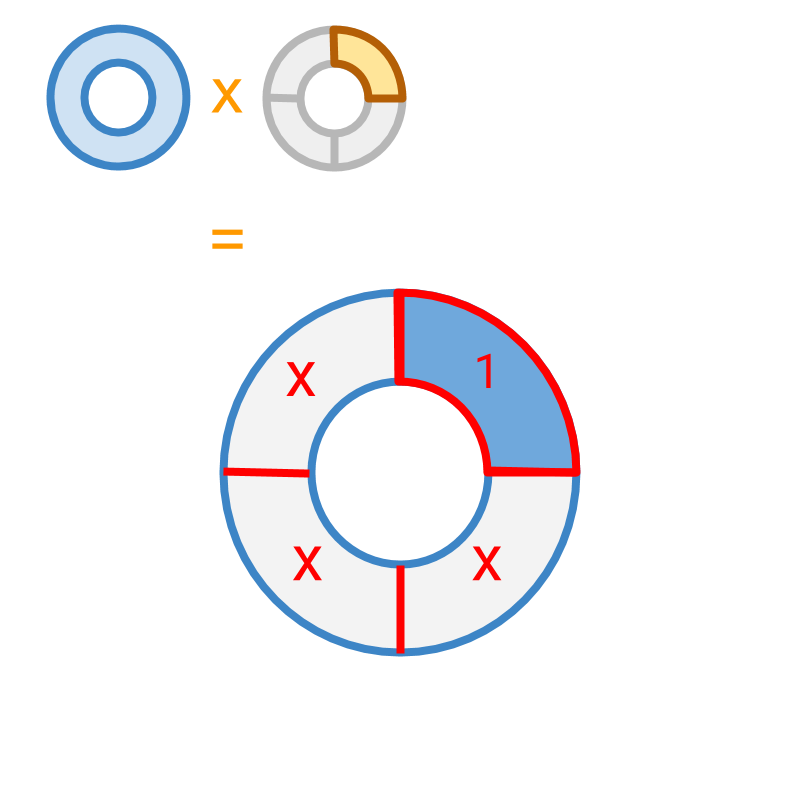The multiplication $1×\frac{1}{4}$$1 \times \frac{1}{4}$ is illustrated.

The multiplicand is divided into $4$$4$ parts (which is the place value of the multiplier) and product is calculated by selecting $1$$1$ part (which is the numerator of the multiplier). The product is $\frac{1}{4}$$\frac{1}{4}$.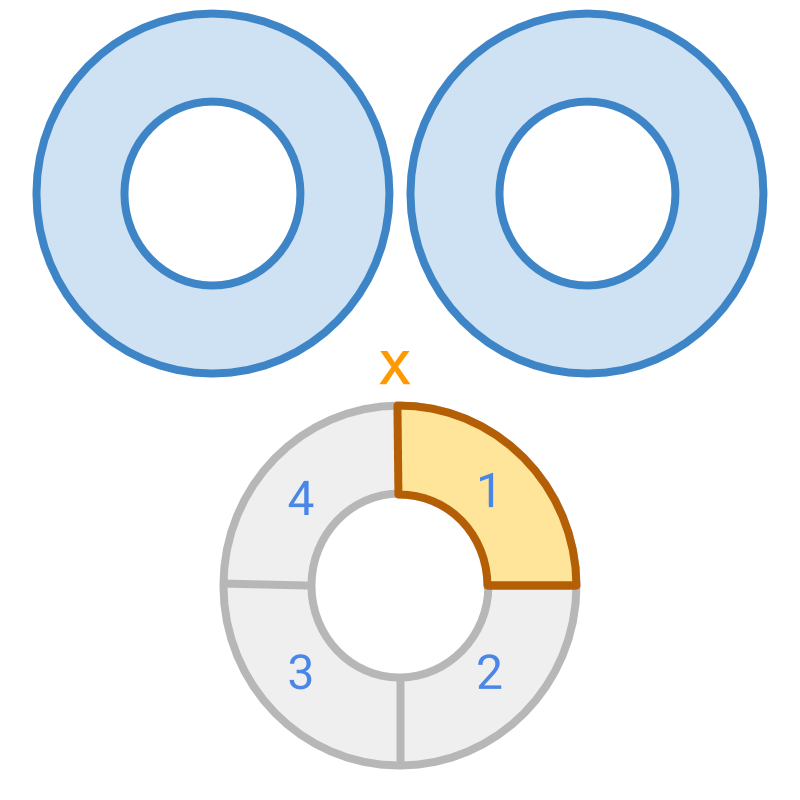Multiplication by a fraction : Consider $2×\frac{1}{4}$$2 \times \frac{1}{4}$
Multiplicand $2$$2$ is multiplied by a multiplier $\frac{1}{4}$$\frac{1}{4}$.
The multiplication process is illustrated in the next page.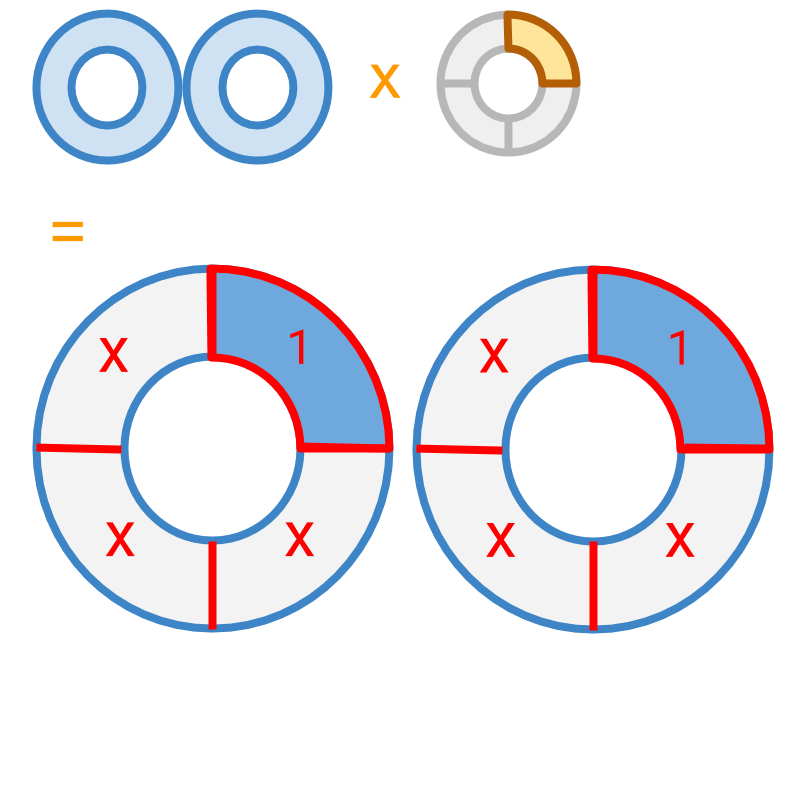The multiplication $2×\frac{1}{4}$$2 \times \frac{1}{4}$ is illustrated.

The multiplicand is divided into $4$$4$ parts (which is the place value of the multiplier) and product is calculated by selecting $1$$1$ part from each (which is the numerator of the multiplier).

The value of one piece with respect to a whole is $\frac{1}{4}$$\frac{1}{4}$ and $2$$2$ pieces are taken. That is, $2\phantom{\rule{1ex}{0ex}}\text{pieces of}\phantom{\rule{1ex}{0ex}}\frac{1}{4}=\frac{2}{4}$$2 \textrm{\pi e c e s o f} \frac{1}{4} = \frac{2}{4}$.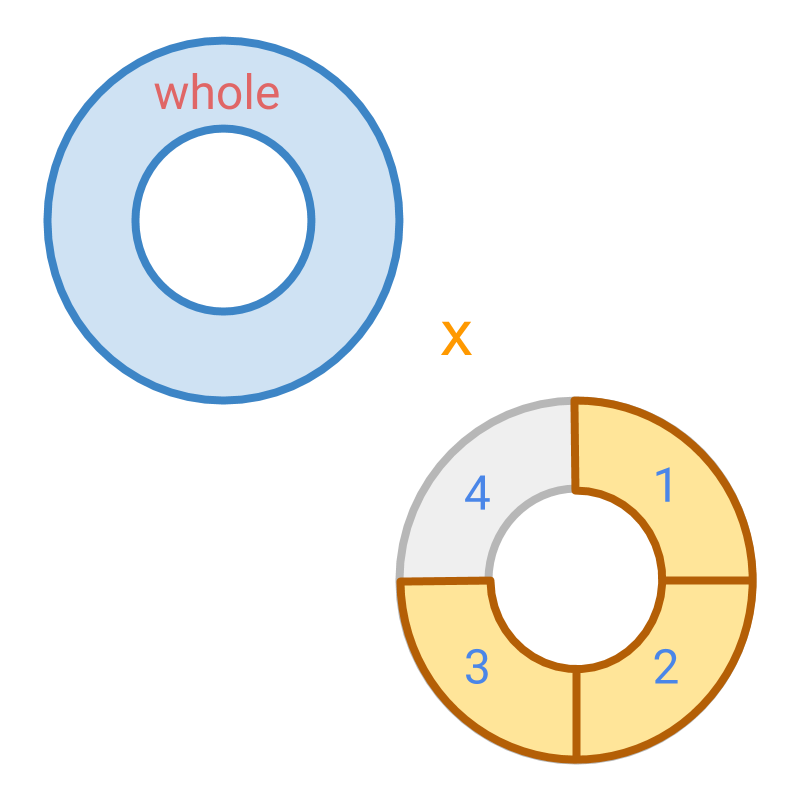Multiplication by a fraction : Consider $1×\frac{3}{4}$$1 \times \frac{3}{4}$
Multiplicand $1$$1$ is multiplied by a multiplier $\frac{3}{4}$$\frac{3}{4}$.
The multiplication process is illustrated in the next page.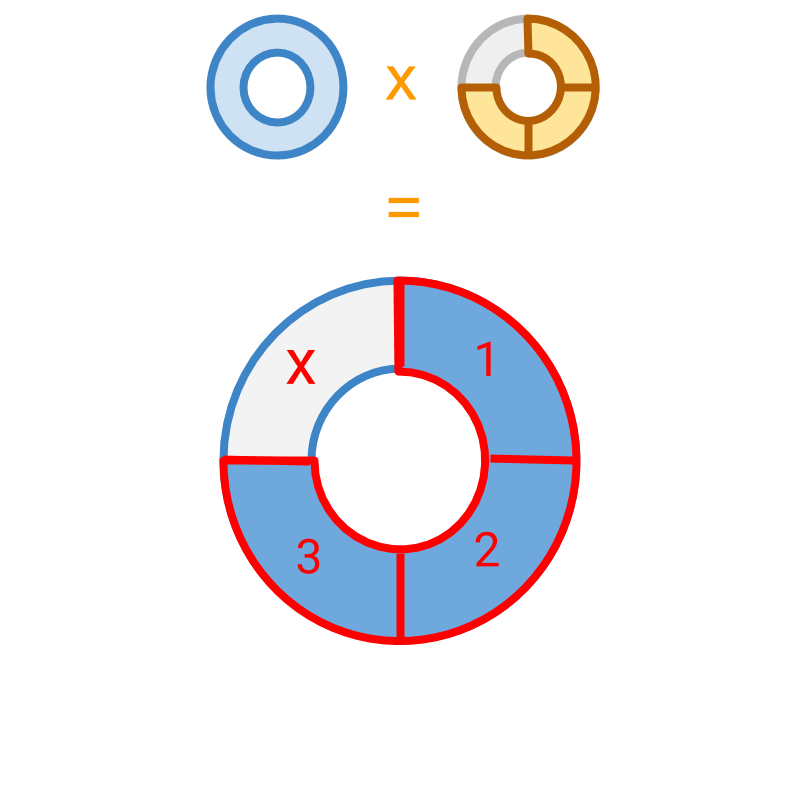The multiplication $1×\frac{3}{4}$$1 \times \frac{3}{4}$ is illustrated.

The multiplicand is divided into $4$$4$ parts (which is the place value of the multiplier) and product is found by selecting $3$$3$ parts (which is the numerator of the multiplier).

The product is $3$$3$ pieces in the place value $\frac{1}{4}$$\frac{1}{4}$ which is $\frac{3}{4}$$\frac{3}{4}$.

fraction by fraction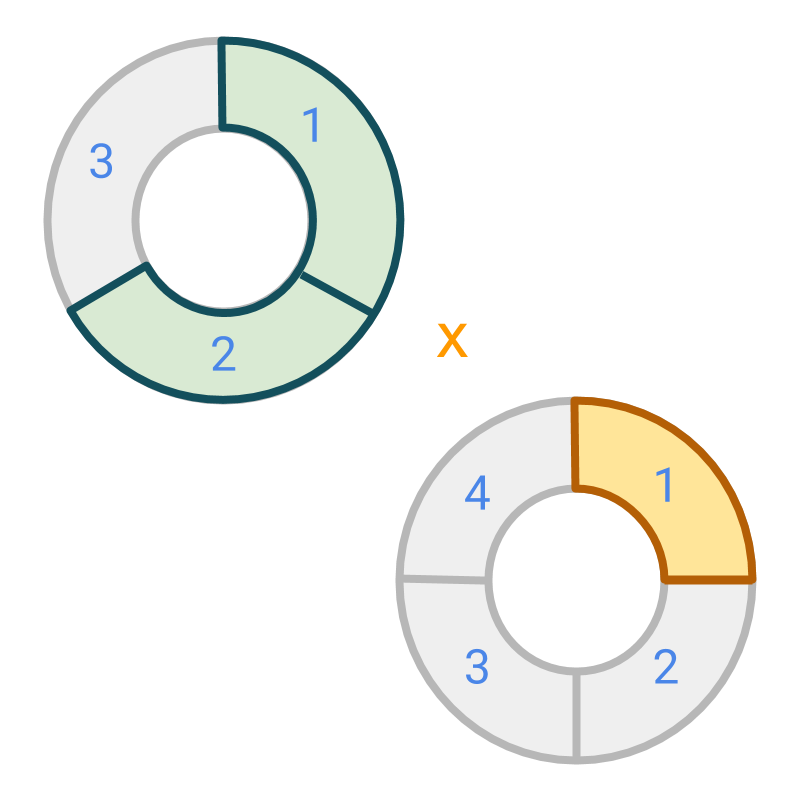Multiplication by a fraction : consider $\frac{2}{3}×\frac{1}{4}$$\frac{2}{3} \times \frac{1}{4}$
Multiplicand $\frac{2}{3}$$\frac{2}{3}$ is multiplied by a multiplier $\frac{1}{4}$$\frac{1}{4}$.
The multiplication process is illustrated in the next page.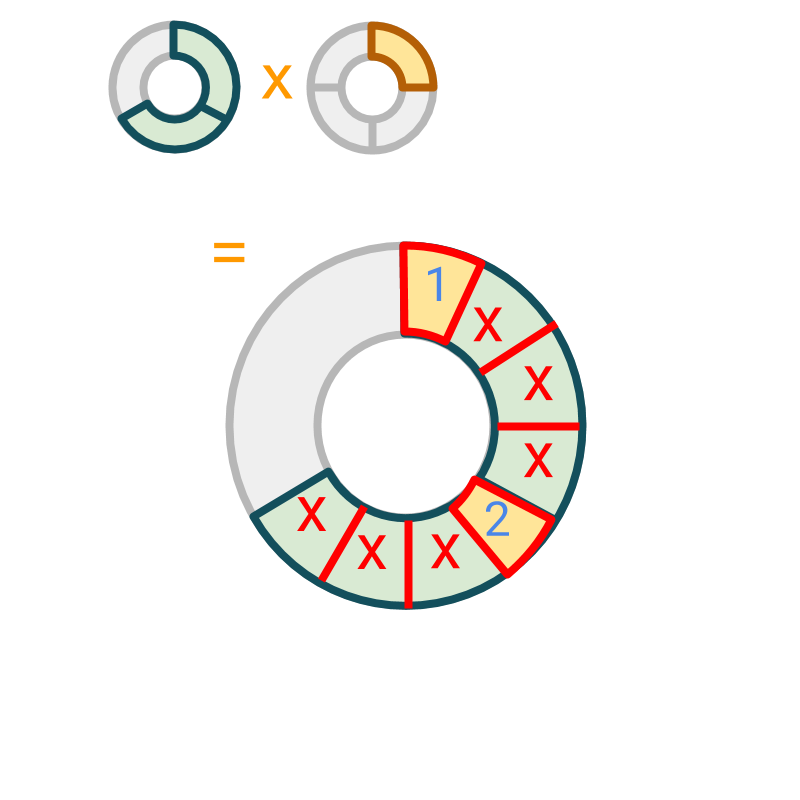The multiplication $\frac{2}{3}×\frac{1}{4}$$\frac{2}{3} \times \frac{1}{4}$ is illustrated.

Each part of multiplicand is divided into $4$$4$ parts (which is the place value of the multiplier) and product is found by selecting $1$$1$ part (which is the numerator of the multiplier) from each.

The product is $2$$2$ pieces in the place value $\frac{1}{12}$$\frac{1}{12}$ which is $\frac{2}{12}$$\frac{2}{12}$.

$\frac{2}{12}$$\frac{2}{12}$ can be simplified to $\frac{1}{6}$$\frac{1}{6}$.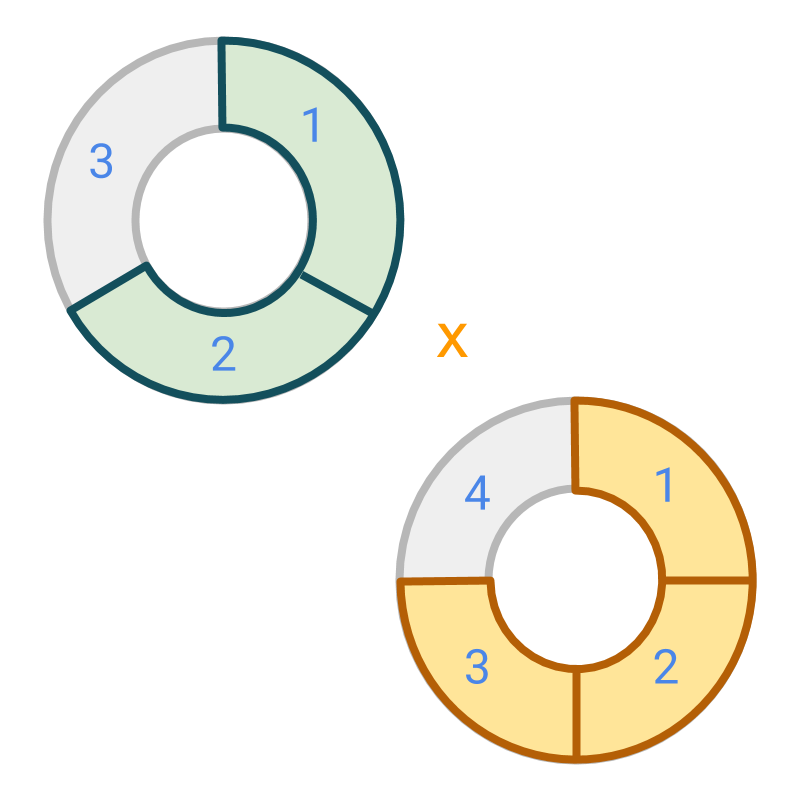Multiplication by a fraction : Consider $\frac{2}{3}×\frac{3}{4}$$\frac{2}{3} \times \frac{3}{4}$
Multiplicand $\frac{2}{3}$$\frac{2}{3}$ is multiplied by a multiplier $\frac{3}{4}$$\frac{3}{4}$.
The multiplication process is illustrated in the next page.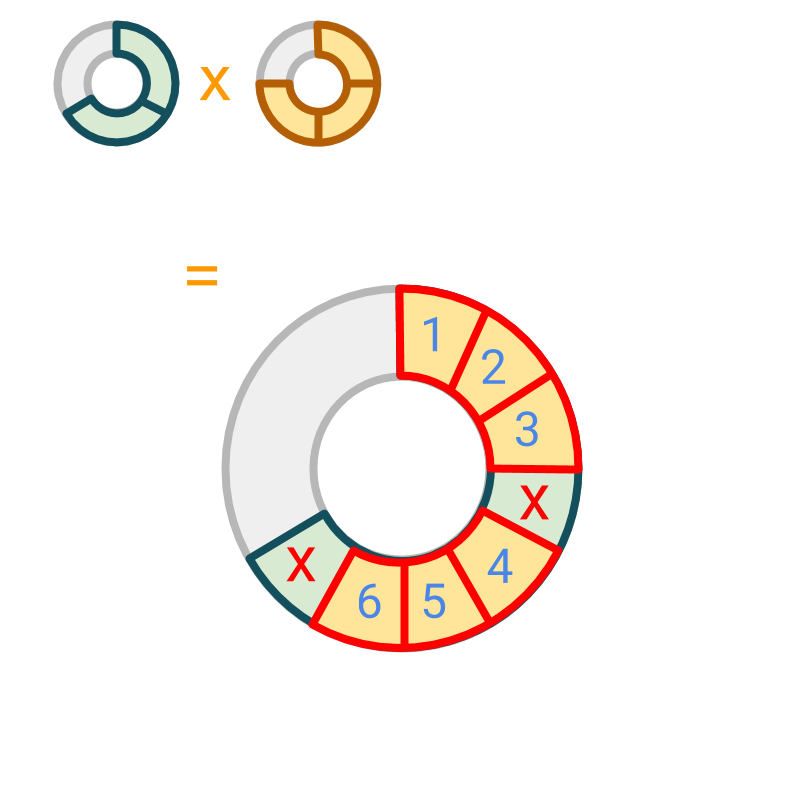The multiplication $\frac{2}{3}×\frac{3}{4}$$\frac{2}{3} \times \frac{3}{4}$ is illustrated.

The each part of multiplicand is divided into $4$$4$ parts (which is the place value of the multiplier) and product is found by selecting $3$$3$ parts (which is the numerator of the multiplier) from each.

The product is $6$$6$ pieces in the place value $\frac{1}{12}$$\frac{1}{12}$ which is $\frac{6}{12}$$\frac{6}{12}$.

$\frac{6}{12}$$\frac{6}{12}$ can be simplified to $\frac{1}{2}$$\frac{1}{2}$.

simplify

Having understood the first principles of multiplication of fractions, the procedural simplification for the same is :

•  Numerators of multiplicand and multiplier are multiplied to numerator of product.

•  Denominators of multiplicand and multiplier are multiplied to denominator of product.

Multiplication is understood in two steps

•  The place value of multiplicand is modified by the place value of multiplier.

•  The given number of multiplicand is multiplied by the given number of multiplier.

Multiplication of Fractions: For two fractions multiplicand $\frac{p}{q}$$\frac{p}{q}$ and the multiplier $\frac{l}{m}$$\frac{l}{m}$
Every $\frac{1}{q}$$\frac{1}{q}$ part of multiplicand is split into $m$$m$ pieces making it $\frac{1}{q×m}$$\frac{1}{q \times m}$ as the modified place value
) From each of $p$$p$ parts of multiplicand $l$$l$ parts make the product making $p×l$$p \times l$ parts
$p×l$$p \times l$ parts in $\frac{1}{q×m}$$\frac{1}{q \times m}$ place value gives the result $\frac{p×l}{q×m}$$\frac{p \times l}{q \times m}$

example

Multiply $\frac{14}{18}×\frac{3}{4}$$\frac{14}{18} \times \frac{3}{4}$.
The answer is '$\frac{7}{12}$$\frac{7}{12}$'

summary

»  $\frac{a}{b}×\frac{c}{d}$$\frac{a}{b} \times \frac{c}{d}$ $=\frac{ac}{bd}$$= \frac{a c}{b d}$
»  $2×\frac{1}{4}$$\textcolor{\mathrm{de} e p s k y b l u e}{2} \times \textcolor{c \mathmr{and} a l}{\frac{1}{4}}$→  $2$$2$ pieces
→  $\frac{1}{4}$$\frac{1}{4}$ of each of those pieces
→  result is count $2$$2$ in place value$\frac{1}{4}$$\frac{1}{4}$
→  $=\frac{2}{4}$$= \frac{2}{4}$

»  $\frac{2}{3}×\frac{3}{4}$$\textcolor{\mathrm{da} r k g r e e n}{\frac{2}{3}} \times \textcolor{c \mathmr{and} a l}{\frac{3}{4}}$→  $2$$\textcolor{\mathrm{da} r k g r e e n}{2}$ pieces in place value $\frac{1}{3}$$\textcolor{\mathrm{da} r k g r e e n}{\frac{1}{3}}$
→  $\frac{3}{4}$$\textcolor{c \mathmr{and} a l}{\frac{3}{4}}$ of each of those pieces
→  result is count $6$$6$ in place value $12$$12$
→  $=\frac{6}{12}=\frac{1}{2}$$= \frac{6}{12} = \frac{1}{2}$

»  Procedural Simplification: Multiply numerators and denominators. Cancel any common factors .
→  $\frac{2}{3}×\frac{3}{4}$$\frac{2}{3} \times \frac{3}{4}$
→  $\frac{2×3}{3×4}$$\frac{2 \times 3}{3 \times 4}$
→  common factors $3$$3$ and $2$$2$ canceled
→  $=\frac{1}{2}$$= \frac{1}{2}$

Outline